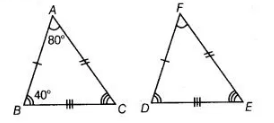# It is given that ΔABC = ΔFDE and AB = 5 cm,`
Question:

It is given that ΔABC = ΔFDE and AB = 5 cm, ∠B = 40° and ∠A = 80°, then Which of the following is true?

(a) DF = 5 cm, ∠F = 60°

(b) DF = 5 cm, ∠E =60°

(c) DE = 5 cm, ∠E = 60°

(d) DE = 5 cm, ∠D = 40°

Solution:

(b) Given, $\triangle A B C \cong \triangle F D E$ and $A B=5 \mathrm{~cm}, \angle B=40^{\circ}, \angle A=80^{\circ}$

Since, $\triangle F D E \cong \triangle A B C$$\therefore \quad D F=A B$ [by CPCT]

$D F=5 \mathrm{~cm}$

and $\angle E=\angle C$ [by CPCT]

$\Rightarrow \quad \angle E=\angle C=180^{\circ}-(\angle A+\angle B)$

[by angle sum property of a $\triangle A B C$ ]

$\Rightarrow \quad \angle E=180^{\circ}-\left(80^{\circ}+40^{\circ}\right)$

$\Rightarrow \quad \angle E=60^{\circ}$